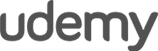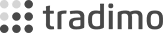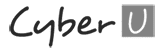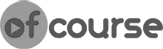Programming Market Exit Conditions – MQL4 for Complete Beginners Tutorial Part 27

In this video we will complete our Forex Robot by adding market exit conditions to it. Specifically, we want to close any existing orders before we the Algorithmic Trading System opens new ones. This way we will make sure that there is only one open order at any given time, therefore limiting our currency risk and exposure.

Feel free to leave me some feedback if you have any questions on the code or if you just like my tutorials!

Source code below

Enrol in the full course here: https://www.forexboat.com/learn-mql4

Code for 4-digit brokers

//+------------------------------------------------------------------+
//| SimpleSystem.mq4 |
//| http://www.forexboat.com |
//+------------------------------------------------------------------+
#property version "1.00"
#property strict

extern int StartHour = 9;
extern int TakeProfit = 40;
extern int StopLoss = 40;
extern double Lots = 1;

void OnTick()
{
static bool IsFirstTick = true;
static int ticket = 0;

if(Hour() == StartHour)
{
if(IsFirstTick == true)
{
IsFirstTick = false;

bool res;
res = OrderSelect(ticket, SELECT_BY_TICKET);
if(res == true)
{
if(OrderCloseTime() == 0)
{
bool res2;
res2 = OrderClose(ticket, Lots, OrderClosePrice(), 10);

if(res2 == false)
{
}
}
}

if(Open < Open[StartHour])
{
ticket = OrderSend(Symbol(), OP_BUY, Lots, Ask, 10, Bid-StopLoss*Point, Bid+TakeProfit*Point, "Set by SimpleSystem");
if(ticket < 0)
{
}
}
else
{
ticket = OrderSend(Symbol(), OP_SELL, Lots, Bid, 10, Ask+StopLoss*Point, Ask-TakeProfit*Point, "Set by SimpleSystem");
if(ticket < 0)
{
}
}

}
}
else
{
IsFirstTick = true;
}

}

Code for 5-digit brokers

//+------------------------------------------------------------------+
//| SimpleSystem.mq4 |
//| http://www.forexboat.com |
//+------------------------------------------------------------------+
#property version "1.00"
#property strict

extern int StartHour = 9;
extern int TakeProfit = 40;
extern int StopLoss = 40;
extern double Lots = 1;

void OnTick()
{
static bool IsFirstTick = true;
static int ticket = 0;

if(Hour() == StartHour)
{
if(IsFirstTick == true)
{
IsFirstTick = false;

bool res;
res = OrderSelect(ticket, SELECT_BY_TICKET);
if(res == true)
{
if(OrderCloseTime() == 0)
{
bool res2;
res2 = OrderClose(ticket, Lots, OrderClosePrice(), 10*10);

if(res2 == false)
{
}
}
}

if(Open < Open[StartHour])
{
//here we are assuming that the TakeProfit and StopLoss are entered in Pips
ticket = OrderSend(Symbol(), OP_BUY, Lots, Ask, 10*10, Bid-StopLoss*Point*10, Bid+TakeProfit*Point*10, "Set by SimpleSystem");
if(ticket < 0)
{
}
}
else
{
//here we are assuming that the TakeProfit and StopLoss are entered in Pips
ticket = OrderSend(Symbol(), OP_SELL, Lots, Bid, 10*10, Ask+StopLoss*Point*10, Ask-TakeProfit*Point*10, "Set by SimpleSystem");
if(ticket < 0)
{
}
}

}
}
else
{
IsFirstTick = true;
}

}

What are you waiting for?

START LEARNING FOREX TODAY!•••••